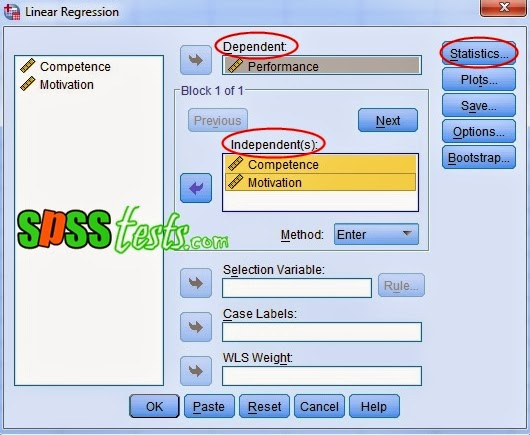# Multicollinearity Test Example Using SPSS

Multicollinearity Test Example Using SPSS | After the normality of the data in the regression model are met, the next step to determine whether there is similarity between the independent variables in a model it is necessary to multicollinearity test. Similarities between the independent variables will result in a very strong correlation. In addition, multicollinearity test done to avoid habits in the decision making process regarding the partial effect of independent variables on the dependent variable. Good regression model should not happen correlation between the independent variables or not happen multicollinearity. Test muticollinearity as a basis the VIF value of multicollinearity test results using SPSS.

Making process in Multicollinearity Test
1. If the VIF value lies between 1-10, then there is no multicollinearity.
2. If the VIF <1 or> 10, then there is multicollinearity.

Case in Multicollinearity Test

A company manager wants to know whether the regression models multicollinearity symptoms or not. Research title is "Influence of Competence and Motivation on Employee Performance". For the manager of the collected data competence, motivation and performance of employees from 40 samples. The research data as shown below.Step By Step to Test Multicollinearity Using SPSS

1. Turn on the SPSS program and select the Variable View, furthermore, in the Name write Competency, Motivation, Performance. Ignore the other options.2. The next step, click the Data View and enter research data in accordance with the variable Competency, Motivation, Performance.3. Next, from the SPSS menu select Analyze-Regression-Linear4. Then a new dialog box will appear with the name of Linear Regression, and then enter the variable competence and motivation to box Independent (s), and then enter the Dependent box to the performance variable, then click the Statistics5. The dialog box appears with the name of Linear Regression: Statistics, provide a check on the collinearity diagnostics, and then click the Continue button6. The last step clicks on Ok to terminate the command, after which it will appear SPSS output, as follows:Interpretation of Test Results Output Multicollinearity

Based on the Coefficients Output - collinearity Statistics, obtained VIF value of 1.812, meaning that the VIF value obtained is between 1 to 10, it can be concluded that there is no multicollinearity symptoms. After the test is completed multicollinearity researchers also should examine whether there was a difference of residual variance observation period to another period of observation by way of heteroscedasticity test.

Next: Test Heteroskidastity Glejser Using SPSS

[Keywords: Multicollinearity Test Example Using SPSS | How To Test Multicollinearity in SPSS with the method VIF | Step By Step To Test Multicollinearity Using SPSS | Tutotial Multicollinearity Test in SPSS with SPSS software version 21]
[Image: Data SPSS Version 21]
[Source: Summarized from various sources]

### 19 Responses to "Multicollinearity Test Example Using SPSS"

1.1.2.3.nice. tq. but one question, if we have control and moderating/mediating variable, should we put all in one model or differently should be tested?

4.nice explanation

5.What is sequence of data analysis of a questionnaire in SPSS?
1. Reliability Test
2. Normality Test
3. Linearity Test
4. Multi Co linearity Test
5. Regression Analysis
is this sequence ok?

Thank you

1.Yes it is good.

6.7.1.8.Supper example!

1.9.10.i wonders the stuff

11.12.If both dependent and independent variable are categorical then how can multicollinearity test be done?

13.please tell me the reference

14.Is this functional for binary logistics regression?

15.very good and well understood